Numbers: Use computational tools and strategies to make estimations and approximations

# Unit 1: Make estimations and approximations

Gill Scott### Unit 1

By the end of this unit you will be able to:

• Use a scientific calculator competently and efficiently.
• Execute algorithms appropriately in calculations.
• Take and record measurements to the degree of accuracy of the instrument.

## What you should know

Before you start this unit, make sure you can:

• Use basic arithmetic operations.
• Work with the decimal system.

## Introduction

A scientific calculator is a useful tool for people working with numbers and doing arithmetical and mathematical calculations. As with all tools, to be effective, users need to know what tasks (or ‘operations’) must be done, what steps to follow and how to use the tools correctly.

Numbers are often combined in a sequence of arithmetical operations (such as addition, subtraction, multiplication, division, raising to powers and finding square, cube and other roots). The order in which calculations are made often affects the answers. For example, is: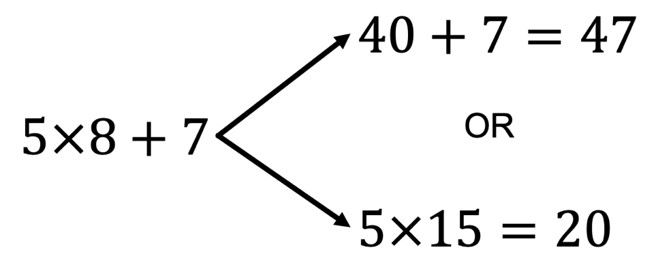In other words, do we multiply five by eight first or do we add eight and seven first?

Although many calculators automatically do the calculations in the correct order, it is necessary to know the rule defining the proper order of priority in which arithmetic operations should be made.

A useful rule to remember is one called BODMAS. BODMAS is a mnemonic (pronounced nemonik). A mnemonic is a system such as a pattern of letters, ideas, or associations which helps you to remember something. BODMAS is a mnemonic for Brackets, Of, Division, Multiplication, Addition, Subtraction.### Take note!

Order of operations – BODMAS

Brackets – work out whatever is inside of any brackets first. You may need to apply the BODMAS order inside brackets as well.

Of – ‘Of’ in an expression means $\scriptsize \times$; or the Orders Of numbers – i.e. Powers, and Square Roots, Cube Roots, etc.

DMDivision and Multiplication (start from left to right)

ASAddition and Subtraction (start from left to right)

Using the BODMAS rule that multiplication must be done before addition, the correct answer to the above calculation is:

$\scriptsize 5\times 8+7=40+7=47$

## Using a scientific calculator

There are many brands of scientific calculators available, each with its own operator manual explaining how to use it. If you lose the operator manual for your calculator, you can download it from the internet. It is important to have a manual for your calculator to refer to as your studies progress and you learn about more advanced mathematical functions.

Two commonly available brands of calculators are CASIO and SHARP. They are shown in Figure 1 to illustrate how scientific calculators can be used in general. YouTube has many calculator tutorials, if ever you are stuck. Here are two you might want to watch now.

How To Use Scientific Calculators (Duration: 3.11)Using a Scientific Calculator (Duration: 17.12)In Figure 1 you can see that each key on the calculator has a number or operator on its face, as well as functions above it, to the left and sometimes the right. Pressing keys in a particular order enters numbers and functions onto the display screen showing the calculation being made, and giving the answer. The functions of some of the keys that you will need are explained below (some are for CASIO and some for SHARP – check on your calculator to see which is relevant).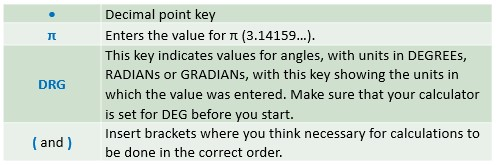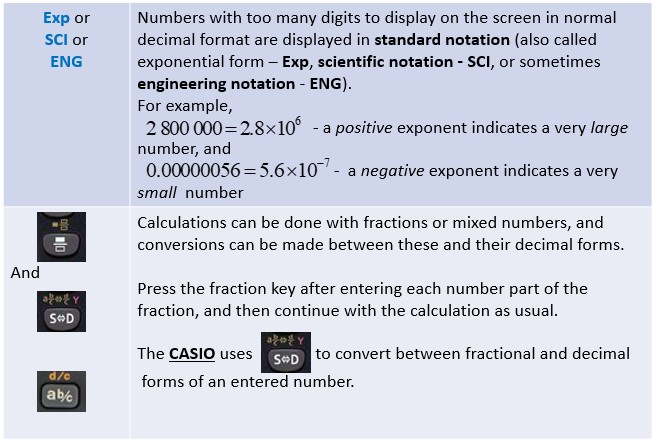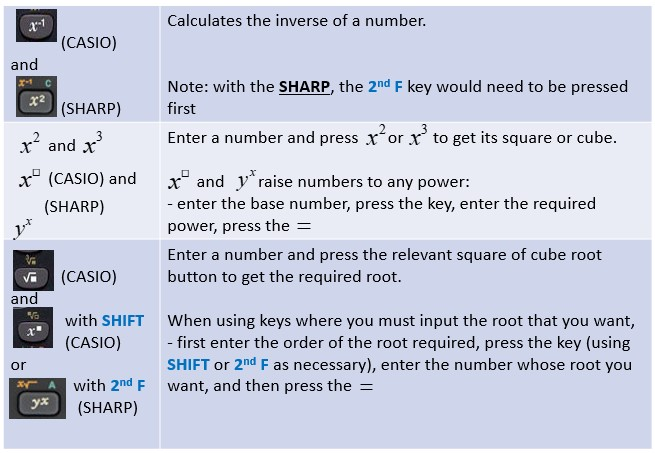### Note

If you would like a short demonstration of calculator basics, watch the video called “Basic Calculator Use”.

Basic Calculator Use (Duration: 16.23)### Example 1.1

Calculate the value of the following expression:

$\scriptsize 11-12\div 4+3\times (6-2)$

Solution

If you enter this string of operations into your calculator as written and then press the key, you should get an answer of $\scriptsize 20$.

Here is the correct order of operations that your calculator will perform.

\scriptsize \begin{align*} & 11-12\div 4+3\times (6-2)\quad \text{The value of the bracket is calculated first}\\ & =11-12\div 4+3\times 4\quad \text{Division is calculated next }\left( {\text{12}\div \text{4}} \right)\\ & =11-3+3\times 4\quad \text{Multiplication is calculated next }\left( {\text{3}\times \text{4}} \right)\\ & =11-3+12\quad \text{Subtraction appears first on the left: calculate }\left( {11-3} \right)\\ & =8+12\quad \text{Addition appears on the right of the subtraction: calculate }\left( {8+12} \right)\\ & =20\end{align*}### Example 1.2

Calculate the value of the following expression:

$\scriptsize {{2.58}^{2}}-{{0.89}^{3}}$

Solution

\scriptsize \begin{align*} 2.58^{2}-0.89^{3} &=6.6564-0.89^{3} &&\text{Enter }2.58 \text{ then press the }x^{2}\text{ key then press the }-\text{ key}\\ &=6.6564-0.704969 &&\text{Enter }0.89 \text{ then press the }x^{\square }\text{ key CASIO }\\ &&&\text{(or for SHARP press the }y^{x}\text{ key) followed by } 3\\ &=5.951431 &&\text{Press the }=\text{ key to get the answer} \end{align*}

Once you are sure of the steps, you need not write them all down, but just carefully press the keys as necessary.### Exercise 1.1

Use your calculator to solve the following expressions:

1. $\scriptsize 3+803.308\times {{10}^{3}}-\sqrt{{64}}$
2. $\scriptsize 603.8\times 21.7-{{52}^{2}}$
3. $\scriptsize {{21.1}^{3}}-\sqrt{{9393.931}}$
4. $\scriptsize 52.785\div 2.3+471.03\times 2.825\times 0.44$

The full solutions are at the end of the unit.

## Approximations

The decimal system is based on powers of $\scriptsize 10$, and each interval between one number and the next can be divided into ten sub-intervals, with $\scriptsize 5$ being the mid-point between the two numbers. Numbers less than $\scriptsize 5$ are closer to the lower number, and numbers greater than $\scriptsize 5$ are closer to the larger number. By agreement, $\scriptsize 5$ is treated as though it is closer to the larger number.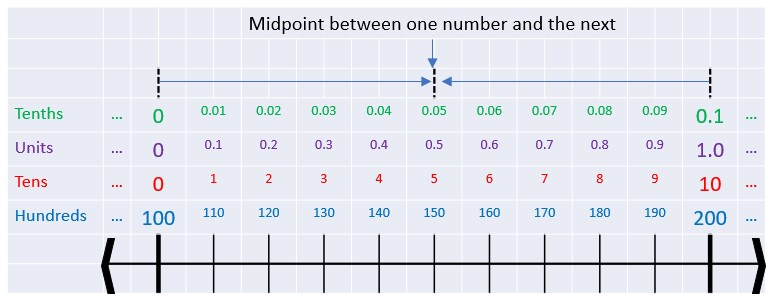This idea is used in approximating numbers, when an answer is required to a specific number of decimal places, or to a given number of ‘significant figures’.

• If the figure to be discarded is $\scriptsize 5$ or more, the previous figure is increased by $\scriptsize 1$.
• If the figure to be discarded is less than $\scriptsize 5$, the previous figure is not changed.

If an answer must be correct to a given number of decimal places, that number of figures must be given after the decimal point.### Example 1.3

1. Round $\scriptsize 0.07823\text{ off to 3 decimal places}$.
2. Round $\scriptsize 0.07823\text{ off to 2 decimal places}$.
3. Round $\scriptsize 0.07823\text{ off to 1 decimal place}$.
4. Round $\scriptsize 23.492\text{ off to 2 decimal places}$.
5. Round $\scriptsize 23.492\text{ off to 1 decimal place}$.

Solutions

1. $\scriptsize 0.07823=0.078\text{ correct to 3 decimal places}$
We start counting digits from the decimal point to the right. In this case, we keep the first three digits after the decimal point and discard from the fourth digit. Because the first digit to be discarded is $\scriptsize 2$ (less than $\scriptsize 5$) we do not need to change the value of the third digit.
2. $\scriptsize 0.07823=0.08\text{ correct to 2 decimal places}$
We start counting digits from the decimal point to the right. In this case, we keep the first two digits following the decimal point, and discard from the third digit. Because the first digit to be discarded is $\scriptsize 8$ (which is greater than or equal to $\scriptsize 5$) we have to round the second digit up i.e. we need to change the $\scriptsize 7$ to an $\scriptsize 8$.
3. $\scriptsize 0.07823=0.1\text{ correct to 1 decimal place}$
We start counting digits from the decimal point to the right. In this case, we keep the first digit only and discard from the second digit. Because the first digit to be discarded is $\scriptsize 7$ (greater than or equal to $\scriptsize 5$) we have to round the first digit up i.e. we need to change the $\scriptsize 0$ into a $\scriptsize 1$.
4.  and 5.
\scriptsize \begin{align*}23.492 & =23.49\text{ correct to 2 decimal places}\\ & =23.5\text{ correct to 1 decimal place}\end{align*}### Example 1.4

1. Solve $\scriptsize 27.4144\div 6.4$.
2. Solve $\scriptsize 27.4144\div 6.4\text{ correct to 3 decimal places}$.
3. Solve $\scriptsize 27.4144\div 6.4\text{ correct to 2 decimal places}$.
4. Solve $\scriptsize 27.4144\div 6.4\text{ correct to 1 decimal place}$.

Solutions

1. $\scriptsize 27.4144\div 6.4=4.2835\text{ }$ The answer is exact, to $\scriptsize 4$ decimal places.
2. .
\scriptsize \begin{align*}27.4144\div 6.4 & =4.2835\\ & =4.284\text{ correct to 3 decimal places}\end{align*}
We keep the first three digits after the decimal point, and discard the fourth digit. Because the digit being discarded ($\scriptsize 5$ in this case) is greater than or equal to $\scriptsize 5$, we round the third digit up i.e. we change the $\scriptsize 3$ into a $\scriptsize 4$.
3. .
\scriptsize \begin{align*}27.4144\div 6.4 & =4.2835\\ & =4.28\text{ correct to 2 decimal places}\end{align*}
We keep the first two digits after the decimal point, and discard the third digit. Because the digit being discarded ($\scriptsize 3$ in this case) is less than $\scriptsize 5$, we keep the second digit the same i.e. $\scriptsize 8$.
4. .
\scriptsize \begin{align*}27.4144\div 6.4 & =4.2835\\ & =4.3\text{ correct to 1 decimal place}\end{align*}
We keep the first digit after the decimal point, and discard from the second digit. Because the first digit being discarded ($\scriptsize 8$ in this case) is greater than or equal to $\scriptsize 5$, we round the first digit up i.e we change the $\scriptsize 2$ into a $\scriptsize 3$.

### Note

All non-zero digits are significant. Zeros that are between other significant figures are significant and must be kept.

\scriptsize \begin{align*}4204\quad 4\text{ significant figures}\\32.002\quad 5\text{ significant figures}\\50.90602\quad 7\text{ significant figures}\end{align*}

Zeros that come before all non-zero significant figures are not significant. The leading zeros serve to place the decimal point correctly.

\scriptsize \begin{align*}0.23\quad 2\text{ significant figures}\\0.00023\quad 2\text{ significant figures}\\0.00203\quad 3\text{ significant figures}\end{align*}

Zeros that follow non-zero digits are not significant unless the decimal point is indicated:

\scriptsize \begin{align*} & 150\text{ is correct to the tens digit: it has 2 significant figures}\text{. But }\\ & \text{150}\text{.0 is correct to the tenths digit}\text{. it has 0 units and 0 tenths:}\text{ it has 4 significant figures}\end{align*}

\scriptsize \begin{align*}43\ 000\quad 2\text{ significant figures}\\43.000\quad 5\text{ significant figures}\\43\ 000\ 564\ 001\quad 11\text{ significant figures}\end{align*}### Example 1.5

1. Write $\scriptsize 27\text{ }283\text{ correct to 4 significant figures}$.
2. Write $\scriptsize 27\text{ }283\text{ correct to 3 significant figures}$.
3. Write $\scriptsize 27\text{ }283\text{ correct to 2 significant figures}$.
4. Write $\scriptsize 0.0887\text{ correct to 2 significant figures}$.
5. Write $\scriptsize 0.0887\text{ correct to 1 significant figure}$.
6. Write $\scriptsize 36.7504\text{ correct to 5 significant figures}$.
7. Write $\scriptsize 36.7504\text{ correct to 3 significant figures}$.

Solutions

1. $\scriptsize 27\text{ }283=27\text{ }280\text{ correct to 4 significant figures}$
Here we need to replace the units digit with a zero to keep the decimal point in its proper position. If we did not do this then we would have changed the whole value of the number by essentially dividing it by $\scriptsize 10$.
2. $\scriptsize 27\text{ }283=27\text{ }300\text{ correct to 3 significant figures}$
Here we use two zeros to keep the decimal point in its proper position.
3. $\scriptsize 27\text{ }283=27\text{ }000\text{ correct to 2 significant figures}$
4.  and 5.
\scriptsize \begin{align*}0.0887 & =0.089\text{ correct to 2 significant figures}\\ & =0.09\text{ correct to 1 significant figure}\end{align*}
1.  and 7.
\scriptsize \begin{align*}36.7504 & =36.750\text{ correct to 5 significant figures}\\ & =36.8\text{ correct to 3 significant figures}\end{align*}### Exercise 1.2

1. Give the following numbers correct to the number of significant figures stated:
1. $\scriptsize 32.86583$
1. to $\scriptsize 6$ significant figures
2. to $\scriptsize 4$ significant figures
3. to $\scriptsize 2$ significant figures
2. $\scriptsize 0.0047582$
1. to $\scriptsize 4$ significant figures
2. to $\scriptsize 3$ significant figures
3. to $\scriptsize 2$ significant figures
3. $\scriptsize 4.97848$
1. to $\scriptsize 5$ significant figures
2. to $\scriptsize 3$ significant figures
3. to $\scriptsize 1$ significant figure
4. $\scriptsize 21.987$
1. to $\scriptsize 2$ significant figures
2. to $\scriptsize 4$ significant figures
3. to $\scriptsize 6$ significant figures
5. $\scriptsize 62.602$
1. to $\scriptsize 4$ significant figures
2. to $\scriptsize 6$ significant figures
6. $\scriptsize \text{32 638 319}$
1. to $\scriptsize 5$ significant figures
2. to $\scriptsize 2$ significant figures
3. to $\scriptsize 10$ significant figures
7. $\scriptsize 4.14976$
1. to $\scriptsize 5$ significant figures
2. to $\scriptsize 4$ significant figures
3. to $\scriptsize 3$ significant figures
2. Give the following numbers correct to the number of decimal places stated:
1. $\scriptsize 5.14987$
1. to $\scriptsize 4$ decimal places
2. to $\scriptsize 3$ decimal places
3. to $\scriptsize 2$ decimal places
2. $\scriptsize 35.285$
1. to $\scriptsize 2$ decimal places
2. to $\scriptsize 1$ decimal place
3. $\scriptsize 0.004977$
1. to $\scriptsize 5$ decimal places
2. to $\scriptsize 4$ decimal places
3. to $\scriptsize 3$ decimal places
4. $\scriptsize 8.4076$
1. to $\scriptsize 3$ decimal places
2. to $\scriptsize 2$ decimal places
3. to $\scriptsize 1$ decimal place
3. Find the value of:
1. $\scriptsize 18.76\div 14.3$ correct to $\scriptsize 2$ decimal places
2. $\scriptsize 0.0396\div 2.51$ correct to $\scriptsize 2$ significant figures
3. $\scriptsize 7.21\div 0.038$ correct to $\scriptsize 3$ significant figures
4. $\scriptsize 5.13\times 7.34$ correct to $\scriptsize 2$ decimal places
5. $\scriptsize 27\times 13\div 17$ correct to $\scriptsize 3$ significant figures

The full solutions are at the end of the unit.

## Estimation

Estimating the answer to a calculation is useful to check that you have not made a mistake in entering it into your calculator. Estimation is particularly useful to check that you have not misplaced the decimal point.### Example 1.6

1. $\scriptsize 0.34\times 0.62$
2. $\scriptsize 213.8\div 39.4$
3. $\scriptsize \displaystyle \frac{{8.198\times 19.56\times 30.82\times 0.198}}{{6.52\times 3.58\times 0.823}}$

Solutions

1. $\scriptsize 0.34\times 0.62$
For a rough estimate, we could use $\scriptsize 0.3$ and $\scriptsize 0.6$.
\scriptsize \begin{align*} & \text{Estimated product = 0}\text{.3}\times \text{0}\text{.6 = 0}\text{.18}\\ & \text{Correct product = 0}\text{.34}\times \text{0}\text{.62 = 0}\text{.2108}\end{align*}
The estimate shows that the answer of $\scriptsize 0.2108$ is correct and that it is not $\scriptsize 2.108$ or $\scriptsize 0.02108$.
2. $\scriptsize 213.8\div 39.4$
For a rough estimate we could use $\scriptsize 200$ and $\scriptsize 40$.
\scriptsize \begin{align*} & \text{Estimated answer = }200\div 40\text{ = 5}\\ & \text{Correct answer }=\text{ }213.8\div 39.4\text{ }\\ & \text{= }5.426\text{ (correct to 3 decimal places)}\end{align*}
Note that the estimate and the correct answer are of the same order.
3. $\scriptsize \displaystyle \frac{{8.198\times 19.56\times 30.82\times 0.198}}{{6.52\times 3.58\times 0.823}}$
Estimated as $\scriptsize \text{= }\displaystyle \frac{{8\times 20\times 30\times 0.2}}{{6\times 4\times 1}}=40$
Correct answer $\scriptsize \text{= 50}\text{.938 (correct to 3 decimal places)}$
The estimate shows that the answer should be $\scriptsize 50.938$, and not $\scriptsize 509.38$ or $\scriptsize 5.0938$.### Exercise 1.3

1. $\scriptsize 32.7\times 0.259$
2. $\scriptsize 0.682\times 0.097\times 2.38$
3. $\scriptsize 78.41\div 23.78$
4. $\scriptsize 0.059\div 0.00268$
5. $\scriptsize \displaystyle \frac{{0.728\times 0.00625}}{{0.0281}}$
6. $\scriptsize \displaystyle \frac{{27.5\times 30.52}}{{11.3\times 2.73}}$

The full solutions are at the end of the unit.

## Summary

In this unit you have learnt the following:

• How to use the basic functions of a scientific calculator.
• How to apply the order of operations when doing calculations.
• How to approximate and round off in the decimal system, to give answers correct to a given number of significant figures, and a given number of decimal places.
• How to use estimation to check calculations.

# Unit 1: Assessment

#### Suggested time to complete: $\scriptsize 10$ minutes

1. Use your calculator to give the answers to the following, correct to $\scriptsize 3$ decimal places.
1. $\scriptsize 27+{{162.51}^{2}}-{{24}^{3}}$
2. $\scriptsize \sqrt{{249.35}}-36.55\times 0.52+{{12.8}^{2}}$
3. $\scriptsize 13.44\times 0.864+12.55\div 3.2-\sqrt{{36}}$
4. $\scriptsize (\sqrt{{125}}+\sqrt{{128}})\times 12.4$
2. Use your calculator to give the answers to the following, correct to $\scriptsize 3$ significant figures.
1. $\scriptsize {{384.6}^{2}}\times \sqrt{{0.925}}+{{(12.35\div 7.57)}^{3}}$
2. $\scriptsize \sqrt{{0.4388}}\div 12.765-265.22\times {{0.08549}^{2}}$

The full solutions are at the end of the unit.

# Unit 1: Solutions

### Exercise 1.1

1. $\scriptsize \text{803 303}$
2. $\scriptsize \text{10 398}\text{.46}$
3. $\scriptsize 9\text{ 372}\text{.831}$
4. $\scriptsize 22.95$

Back to Exercise 1.1

### Exercise 1.2

1. .
1. .
1. $\scriptsize 32.8658$
2. $\scriptsize 32.87$
3. $\scriptsize 33$
2. .
1. $\scriptsize 0.004758$
2. $\scriptsize 0.00476$
3. $\scriptsize 0.0048$
3. .
1. $\scriptsize 4.9785$
2. $\scriptsize 4.98$
3. $\scriptsize 5.0$
4. .
1. $\scriptsize 22$
2. $\scriptsize 21.99$
3. $\scriptsize 21.9870$
5. .
1. $\scriptsize 62.60$
2. $\scriptsize 62.6020$
6. .
1. $\scriptsize \text{32 638 000}$
2. $\scriptsize \text{33 000 000}$
3. $\scriptsize 32\text{ }638\text{ }310.00$
7. .
1. $\scriptsize 4.1498$
2. $\scriptsize 4.150$
3. $\scriptsize 4.15$
2. .
1. .
1. $\scriptsize 5.1499$
2. $\scriptsize 5.150$
3. $\scriptsize 5.15$
2. .
1. $\scriptsize 35.29$
2. $\scriptsize 35.3$
3. .
1. $\scriptsize 0.00498$
2. $\scriptsize 0.0050$
3. $\scriptsize 0.005$
4. .
1. $\scriptsize 8.408$
2. $\scriptsize 8.41$
3. $\scriptsize 8.4$
3. .
1. $\scriptsize 1.31$
2. $\scriptsize 0.016$
3. $\scriptsize 190$
4. $\scriptsize 37.65$
5. $\scriptsize 20.6$

Back to Exercise 1.2

### Exercise 1.3

1. .
\scriptsize \begin{align*} & 32.7\times 0.259\\ & \text{Estimated as 30}\times 0.3=9\\ & \text{Correct answer}=8.469\text{ correct to 3 decimal places}\end{align*}
2. .
\scriptsize \begin{align*} & 0.682\times 0.097\times 2.38\\ & \text{Estimated as }1\times 0.1\times 2=0.2\\ & \text{Correct answer =}0.157\text{ correct to 3 decimal places}\end{align*}
3. .
\scriptsize \begin{align*} & 78.41\div 23.78\\ & \text{Estimated as }80\div 20=4\\ & \text{Correct answer }=3.297\text{ correct to 3 decimal places}\end{align*}
4. .
\scriptsize \begin{align*} & 0.059\div 0.00268\\ & \text{Estimated as 0}\text{.06}\div \text{0}\text{.003 = 20}\\ & \text{Correct answer = 22}\text{.015 correct to 3 decimal places}\end{align*}
5. .
\scriptsize \begin{align*} & \displaystyle \frac{{0.728\times 0.00625}}{{0.0281}}\\ & \text{Estimated as }\displaystyle \frac{{1\times 0.006}}{{0.03}}=0.2\\ & \text{Correct answer = 0}\text{.162 correct to 3 decimal places}\end{align*}
6. .
\scriptsize \begin{align*} & \displaystyle \frac{{27.5\times 30.52}}{{11.3\times 2.73}}\\ & \text{Estimated as }\displaystyle \frac{{30\times 30}}{{10\times 3}}=30\\ & \text{Correct answer is = 27}\text{.207 correct to 3 decimal places}\end{align*}

Back to Exercise 1.3

### Unit 1: Assessment

The answers to the steps in the calculations are given to help identify any mistakes that may have been made.

1. .
1. .
\scriptsize \begin{align*}27+{{162.51}^{2}}-{{24}^{3}} & =27+26409.5001-13824\\ & =12612.500\text{ correct to 3 decimal places}\end{align*}
2. .
\scriptsize \begin{align*} & \sqrt{{249.35}}-36.55\times 0.52+{{12.8}^{2}}\\ & =15.7908-19.006+163.84\\ & =160.625\text{ correct to 3 decimal places}\end{align*}
3. .
\scriptsize \begin{align*} & 13.44\times 0.864+12.55\div 3.2-\sqrt{{36}}\\ & =11.6122+3.9219-3.3019\\ & =12.232\text{ correct to 3 decimal places}\end{align*}
4. .
\scriptsize \begin{align*} & (\sqrt{{125}}+\sqrt{{128}})\times 12.4\\ & =(11.1803+5.0397)\times 12.4\\ & =162200\times 12.4\\ & =201.128\text{ correct to 3 decimal places}\end{align*}
2. .
1. .
\scriptsize \begin{align*} & {{384.6}^{2}}\times \sqrt{{0.925}}+{{(12.35\div 7.57)}^{3}}\\ & =147917.16\times 0.96177+{{(1.63144)}^{3}}\\ & =142262.287+4.3422\\ & =142266.6292\\ & =142000\text{ correct to 3 significant figures}\end{align*}
2. .
\scriptsize \begin{align*} & \sqrt{{0.4388}}\div 120.765-5.2264\times {{0.008549}^{2}}\\ & =0.7599\div 120.765-5.2264\times 0.000073085\\ & =0.006292-0.000381973\\ & =0.0059104\\ & =0.00591\text{ correct to 3 significant figures}\end{align*}

Back to Unit 1: Assessment